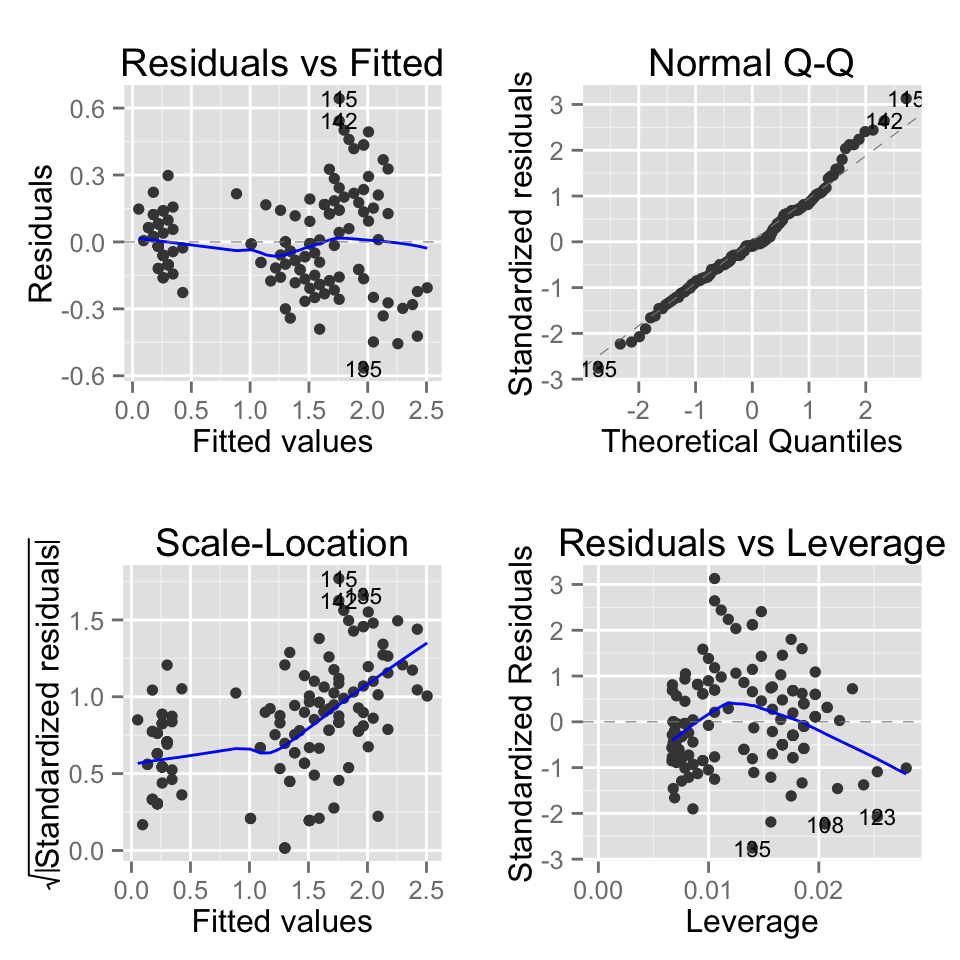This document explains plotting diagnostics for LM and GLM using `{ggplot2}` and `{ggfortify}`.

# Installation

First, install `ggfortify` from CRAN.

``install.packages('ggfortify')``

# Plotting Diagnostics for Linear Models

`{ggfortify}` let `{ggplot2}` know how to interpret `lm` objects. After loading `{ggfortify}`, you can use `ggplot2::autoplot` function for `lm` objects.

``````library(ggfortify)
autoplot(lm(Petal.Width ~ Petal.Length, data = iris), label.size = 3)``````You can select desired plot by `which` option as the same manner as standard `plot`. Also, `ncol` and `nrow` allows you to specify the number of subplot columns and rows.

``````par(mfrow = c(1, 2))
m <- lm(Petal.Width ~ Petal.Length, data = iris)

autoplot(m, which = 1:6, ncol = 3, label.size = 3)``````